# 输入表单入门

• 创建对象：
• Beginner
Intermediate
Developer

## 编辑输入表单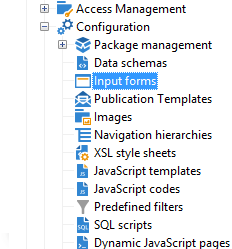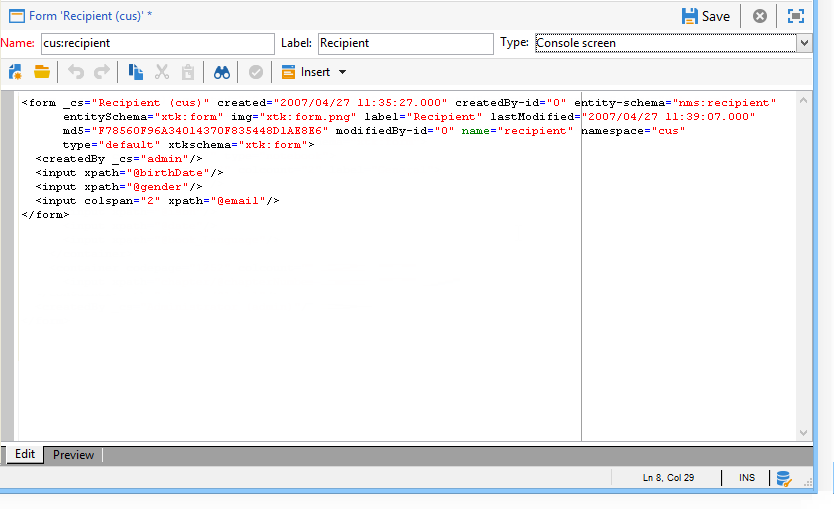## 窗体结构

``````<form name="form_name" namespace="name_space">
...
</form>
``````

``````<srcSchema name="recipient" namespace="cus">
<enumeration name="gender" basetype="byte">
<value name="unknown" label="Not specified" value="0"/>
<value name="male" label="Male" value="1"/>
<value name="female" label="Female" value="2"/>
</enumeration>

<element name="recipient">
<attribute name="email" type="string" length="80" label="Email" desc="E-mail address of recipient"/>
<attribute name="birthDate" type="datetime" label="Date"/>
<attribute name="gender" type="byte" label="Gender" enum="gender"/>
</element>
</srcSchema>
````````````<form name="recipient" namespace="cus">
<input xpath="@gender"/>
<input xpath="@birthDate"/>
<input xpath="@email"/>
</form>
``````

`<input label="E-mail address" xpath="@name" />`所有表单属性都列在 Campaign Classicv7文档.

## 格式化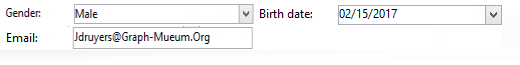``````<form name="recipient" namespace="cus">
<container colcount="2">
<input xpath="@gender"/>
<input xpath="@birthDate"/>
<input xpath="@email"/>
</container>
</form>
``````

`<container>` 元素和 colcount 属性允许将子控件的显示强制到两列上。

colspan 控件的属性按其值中输入的列数来扩展控件：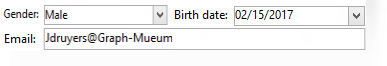``````<form name="recipient" namespace="cus">
<container colcount="2">
<input xpath="@gender"/>
<input xpath="@birthDate"/>
<input xpath="@email" colspan="2"/>
</container>
</form>
``````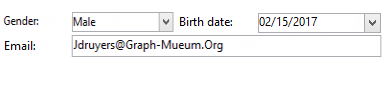``````<form name="recipient" namespace="cus">
<container colcount="2" type="frame" label="General">
<input xpath="@gender"/>
<input xpath="@birthDate"/>
<input xpath="@email" colspan="2"/>
</container>
</form>
``````

A `<static>` 元素可用于设置输入表单的格式：``````<form name="recipient" namespace="cus">
<static type="separator" colspan="2" label="General"/>
<input xpath="@gender"/>
<input xpath="@birthDate"/>
<input xpath="@email" colspan="2"/>
<static type="help" label="General information about recipient with date of birth, gender, and e-mail address." colspan="2"/>
</form>
``````

`<static>` 标记和 分隔符 type用于添加分隔条，其标签包含在 标签 属性。

## 使用容器

xpath 上的属性 `<container>` 允许您简化子控件的引用。 然后，控件的引用将相对于父项 `<container>` 父级。

``````<container colcount="2">
<input xpath="location/@zipCode"/>
<input xpath="location/@city"/>
</container>
``````

``````<container colcount="2" xpath="location">
<input xpath="@zipCode"/>
<input xpath="@city"/>
</container>
``````

### 添加选项卡（笔记本）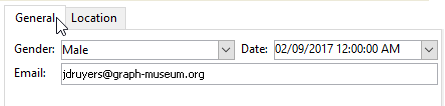``````<container type="notebook">
<container colcount="2" label="General">
<input xpath="@gender"/>
<input xpath="@birthDate"/>
<input xpath="@email" colspan="2"/>
</container>
<container colcount="2" label="Location">
...
</container>
</container>
```````<container style="down" type="notebook"> ... </container>`

### 添加图标（图标框）``````<container type="iconbox">
<container colcount="2" label="General" img="xtk:properties.png">
<input xpath="@gender"/>
<input xpath="@birthDate"/>
<input xpath="@email" colspan="2"/>
</container>
<container colcount="2" label="Location" img="nms:msgfolder.png">
...
</container>
</container>
``````

### 隐藏容器(visibleGroup)

``````<container type="visibleGroup" visibleIf="@gender=1">
...
</container>
<container type="visibleGroup" visibleIf="@gender=2">
...
</container>
``````

• visibleIf="@email='peter.martinezATneeolane.net'"：测试字符串类型数据的相等性。 比较值必须用引号括起来。
• visibleIf="@gender >= 1和@gender！= 2英寸：数字值的条件。
• visibleIf="@boolean1=true或@boolean2=false"：测试布尔字段。

### 条件显示(enabledGroup)

``````<container type="enabledGroup" enabledIf="@gender=1">
...
</container>
<container type="enabledGroup" enabledIf="@gender=2">
...
</container>
``````

``````<element label="Company" name="company" target="cus:company" type="link"/>
``````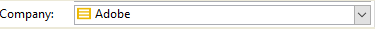``````<input xpath="company"/>
``````

Edit link （放大镜）图标将启动链接元素的编辑表单。 默认情况下，使用的形式是在目标架构的键上推导的。 此 表单 属性允许您强制使用编辑表单的名称（例如“cus：company2”）。

``````<input xpath="company">
<sysFilter>
<condition expr="[location/@city] =  'Newton"/>
</sysFilter>
</input>
``````

``````<input xpath="company">
<orderBy>
<node expr="[location/@zipCode]"/>
</orderBy>
</input>
``````

## 控件属性

• noAutoComplete：禁用提前键入（值为“true”）

• createMode：如果链接不存在，则即时创建该链接。 可能的值包括：

• ：禁用创建。 如果链接不存在，则显示错误消息
• 内嵌：在编辑字段中创建包含内容的链接
• 版本：在链接上显示编辑表单。 验证表单后，数据即会保存（默认模式）
• noZoom：链接上无编辑表单（值为“true”）

• 表单：重载目标元素的编辑表单

``````<element label="Events" name="rcpEvent" target="cus:event" type="link" unbound="true">
...
</element>
``````

`````` <input xpath="rcpEvent" type="linklist">
<input xpath="@label"/>
<input xpath="@date"/>
</input>
``````

Detail 按钮添加时机 zoom="true" 属性完成于 `<input>` 列表的标记：用于启动所选行的编辑表单。

`````` <input xpath="rcpEvent" type="linklist">
<input xpath="@label"/>
<input xpath="@date"/>
<sysFilter>
<condition expr="@type = 1"/>
</sysFilter>
<orderBy>
<node expr="@date" sortDesc="true"/>
</orderBy>
</input>
``````

## 定义关系表

``````<srcSchema name="subscription" namespace="cus">
<element name="service" type="link" target="cus:service" label="Subscription service"/>
</srcSchema>
``````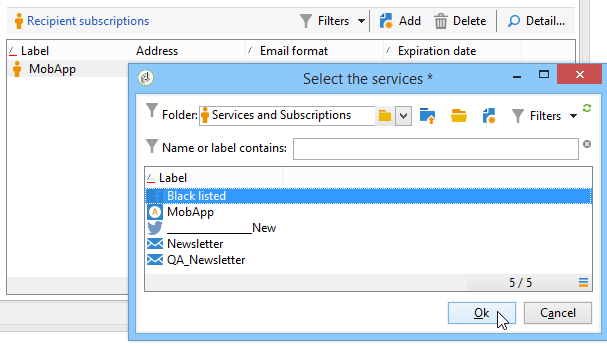``````<input type="linklist" xpath="subscription" xpathChoiceTarget="service" xpathEditTarget="service" zoom="true">
<input xpath="recipient"/>
<input xpath="service"/>
</input>
``````

Xpatchoicetarget 属性允许您从输入的链接启动选择表单。 创建关系表记录将自动更新指向当前收件人和所选服务的链接。

xpathEditTarget 属性允许您在输入的链接上强制编辑所选行。

### 列表属性

• noToolbar：隐藏工具栏（值为“true”）
• toolbarCaption：重载工具栏标签
• toolbarAlign：修改工具栏的垂直或水平几何（可能的值：“垂直”|“水平”）
• img：显示与列表关联的图像
• 表单：重载目标元素的编辑表单
• 缩放：添加 Zoom 按钮以编辑目标元素
• xpathEditTarget：在输入的链接上设置编辑
• Xpatchoicetarget：此外，会在输入的链接上启动选择表单

## 添加列列表

``````<input xpath="rcpEvent" type="list">
<input xpath="@label"/>
<input xpath="@date"/>
</input>
``````

``````<input nolabel="true" toolbarCaption="List of events" type="list" xpath="rcpEvent" zoom="true">
<input xpath="@label"/>
<input xpath="@date"/>
</input>
``````

toolbarCaption 属性强制水平对齐工具栏并输入列表上方的标题。

### 启用放大列表

``````<input nolabel="true" toolbarCaption="List of events" type="list" xpath="rcpEvent" zoom="true" zoomOnAdd="true">
<input xpath="@label"/>
<input xpath="@date"/>

<form colcount="2" label="Event">
<input xpath="@label"/>
<input xpath="@date"/>
</form>
</input>
``````

### 列表属性

• noToolbar：隐藏工具栏（值为“true”）
• toolbarCaption：重载工具栏标签
• toolbarAlign：修改工具栏的位置（可能的值： "vertical"|"horizontal"）
• img：显示与列表关联的图像
• 表单：重载目标元素的编辑表单
• 缩放：添加 Zoom 按钮以编辑目标元素
• Xpatchoicetarget：此外，会在输入的链接上启动选择表单

## 添加不可编辑的字段

“性别”字段示例：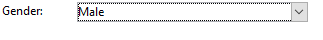``````<value value="@gender"/>
``````

## 添加单选按钮

“性别”字段示例：

``````<input type="RadioButton" xpath="@gender" checkedValue="0" label="Choice 1"/>
<input type="RadioButton" xpath="@gender" checkedValue="1" label="Choice 2"/>
<input type="RadioButton" xpath="@gender" checkedValue="2" label="Choice 3"/>
``````## 添加复选框

``````<input xpath="@boolean1"/>
<input xpath="@field1" type="checkbox" checkedValue="Y"/>
``````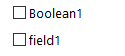``````<input nolabel="true" type="treeEdit">
<container label="Text fields">
<input xpath="@text1"/>
<input xpath="@text2"/>
</container>
<container label="Boolean fields">
<input xpath="@boolean1"/>
<input xpath="@boolean2"/>
</container>
</input>
``````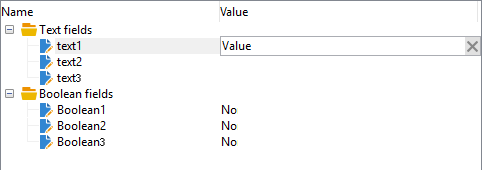## 添加表达式字段

``````<!-- Example: updating the boolean1 field from the value contained in the field with path /tmp/@flag -->
<input expr="Iif([/tmp/@flag]=='On', true, false)" type="expr" xpath="@boolean1"/>
<input expr="[/ignored/@action] == 'FCP'" type="expr" xpath="@launchFCP"/>
``````

## 表单的上下文

### 更新上下文

• `<set expr="'Test'" xpath="/tmp/@test" />`：将“Test”值放置在临时位置/tmp/@test1
• `<set expr="'Test'" xpath="@lastName" />`：使用“Test”值更新“lastName”属性上的实体
• `<set expr="true" xpath="@boolean1" />`：将“boolean1”字段的值设置为“true”
• `<set expr="@lastName" xpath="/tmp/@test" />`：使用“lastName”属性的内容进行更新

``````<form name="recipient" namespace="cus">
<enter>
<set...
</enter>
...
<leave>
<set...
</leave>
</form>
``````

`<enter>``<leave>` 标记可用于 `<container>` （“notebook”和“iconbox”类型）。

### 表达式语言

`<if expr="<expression>" />` 如果验证了表达式，则标记执行在标记下指定的指令：

``````<if expr="([/tmp/@test] == 'Test' or @lastName != 'Doe') and @boolean2 == true">
<set xpath="@boolean1" expr="true"/>
</if>
``````

`<check expr="<condition>" />` 标记与 `<error>` 标记阻止表单验证，如果不满足以下条件，则显示错误消息：

``````<leave>
<check expr="/tmp/@test != ''">
<error>You must populate the 'Test' field!</error>
</check>
</leave>
``````

## 助手（向导）

``````<form type="wizard" name="example" namespace="cus" img="nms:rcpgroup32.png" label="Wizard example" entity-schema="nms:recipient">
<container title="Title of page 1" desc="Long description of page 1">
<input xpath="@lastName"/>
<input xpath="comment"/>
</container>
<container title="Title of page 2" desc="Long description of page 2">
...
</container>
...
</form>
``````

Finish 按钮保存输入的数据并关闭表单。

### SOAP方法

SOAP方法执行可以从填充的 `<leave>` 标签进行标记。

`<soapcall>` 标记包含对方法的调用，并具有以下输入参数：

``````<soapCall name="<name>" service="<schema>">
<param type="<type>" exprIn="<xpath>"/>
...
</soapCall>
``````

• 字符串：字符串
• 布尔型：布尔型
• 字节：8位整数
• ：16位整数
• ：32位整数
• ：16位整数
• 多次：双精度浮点数
• 圆顶元素：元素类型节点

exprIn 属性包含作为参数传递的数据的位置。

``````<leave>
<soapCall name="RegisterGroup" service="nms:recipient">
<param type="DOMElement" exprIn="/tmp/entityList"/>
<param type="DOMElement" exprIn="/tmp/choiceList"/>
<param type="boolean"    exprIn="true"/>
</soapCall>
</leave>
``````